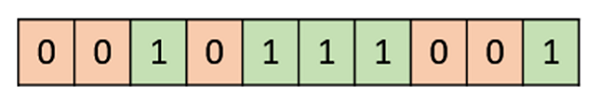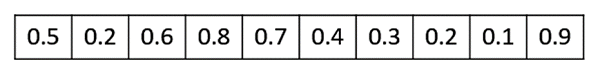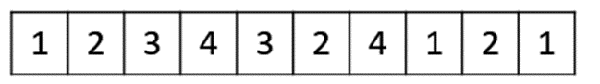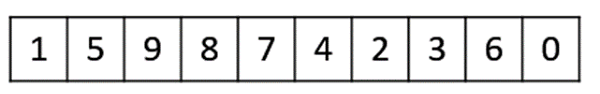Genotype Representation

One of the most important decisions to make while implementing a genetic algorithm is deciding the representation that we will use to represent our solutions. It has been observed that improper representation can lead to poor performance of the GA.

Therefore, choosing a proper representation, having a proper definition of the mappings between the phenotype and genotype spaces is essential for the success of a GA.

In this section, we present some of the most commonly used representations for genetic algorithms. However, representation is highly problem specific and the reader might find that another representation or a mix of the representations mentioned here might suit his/her problem better.

Binary Representation

This is one of the simplest and most widely used representation in GAs. In this type of representation the genotype consists of bit strings.

For some problems when the solution space consists of Boolean decision variables – yes or no, the binary representation is natural. Take for example the 0/1 Knapsack Problem. If there are n items, we can represent a solution by a binary string of n elements, where the xth element tells whether the item x is picked (1) or not (0).For other problems, specifically those dealing with numbers, we can represent the numbers with their binary representation. The problem with this kind of encoding is that different bits have different significance and therefore mutation and crossover operators can have undesired consequences. This can be resolved to some extent by using Gray Coding, as a change in one bit does not have a massive effect on the solution.

Real Valued Representation

For problems where we want to define the genes using continuous rather than discrete variables, the real valued representation is the most natural. The precision of these real valued or floating point numbers is however limited to the computer.Integer Representation

For discrete valued genes, we cannot always limit the solution space to binary ‘yes’ or ‘no’. For example, if we want to encode the four distances – North, South, East and West, we can encode them as {0,1,2,3}. In such cases, integer representation is desirable.Permutation Representation

In many problems, the solution is represented by an order of elements. In such cases permutation representation is the most suited.

A classic example of this representation is the travelling salesman problem (TSP). In this the salesman has to take a tour of all the cities, visiting each city exactly once and come back to the starting city. The total distance of the tour has to be minimized. The solution to this TSP is naturally an ordering or permutation of all the cities and therefore using a permutation representation makes sense for this problem.